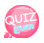# while statement

while means while. It is a loop statement like the for statement. It is used to repeat something.
The syntax is different from the for statement.
The while statement first declares a variable.
After that, we write a while(condition)
Enter the increment value in the statement.
As a source

```\$num = 1;   //Variable declaration
while(\$num <= 10){  //do the following while num is less than or equal to 10
echo "{\$num} output............... {\$a} <br />"; // Do this sentence
\$num++  //Here's the run. When executed, the num value becomes 2, and then back to the while statement, the result is true and the following is repeated.
}```

Now type the output directly from 1 to 10 with the while statement !!!

```<?php
\$num = 1;

while(\$num <= 10){
echo " \$num ";
\$num++;
}
?>```if so!! Now output the odd sum from 1 to 10 using the while statement.

```<?php
echo "Sum of odd numbers from 1 to 10<br />";

\$num = 1;  // Initial value declaration
\$sum = 0;  // Declaration of Variables in Cumulative Totals

while(\$num <= 10){
echo \$num."Cumulative sum to";
\$sum += \$num;
\$num+=2;
echo " = {\$sum} <br />";
}

echo "The cumulative sum of odd numbers 1 through 10 is {\$sum}.<br />";
?>```Declare an initial value of 1 for num.
Then we declare sum to accumulate the sum of odd numbers from 1 to 10.

Set the condition to 10 with the while statement

Accumulate the value of num in \$sum. num is currently 1, so sum is 1.
After that, the value of num is +2. Then num becomes 3.
Going back to while and running as above, 1 3 5 7 9 accumulates in sum.

If you accumulate multiples of 5 from 1 to 100,
Set the condition of the while statement to 100
\$num += 5